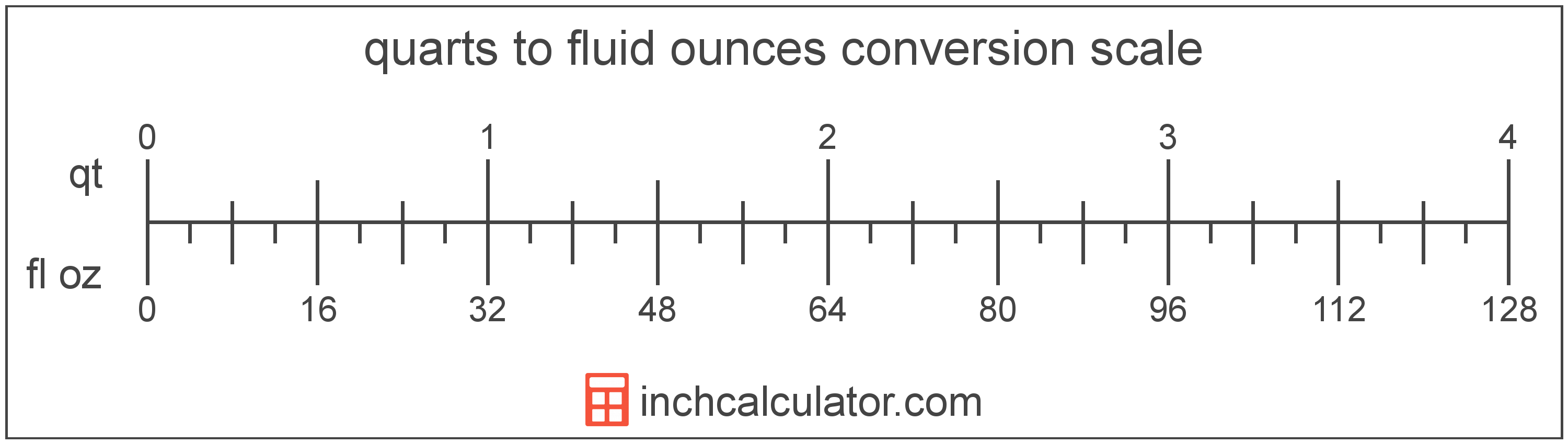# Quarts to Fluid Ounces Converter

Enter the volume in quarts below to get the value converted to fluid ounces.

Results in Fluid Ounces:1 qt = 32 fl oz

Do you want to convert fluid ounces to quarts?

## How to Convert Quarts to Fluid Ounces

To convert a measurement in quarts to a measurement in fluid ounces, multiply the volume by the following conversion ratio: 32 fluid ounces/quart.

Since one quart is equal to 32 fluid ounces, you can use this simple formula to convert:

fluid ounces = quarts × 32

The volume in fluid ounces is equal to the volume in quarts multiplied by 32.

For example, here's how to convert 5 quarts to fluid ounces using the formula above.
fluid ounces = (5 qt × 32) = 160 fl oz### How Many Fluid Ounces Are in a Quart?

There are 32 fluid ounces in a quart, which is why we use this value in the formula above.

1 qt = 32 fl oz

## What Is a Quart?

The US liquid quart is a unit of fluid volume equal to one fourth of a gallon, two pints, or four cups.

The liquid quart should not be confused with the dry quart (US) or the imperial quart, which are different units. However, when someone refers to a volume in quarts, they typically mean the US liquid quart.

The quart is a US customary unit of volume. Quarts can be abbreviated as qt; for example, 1 quart can be written as 1 qt.

## What Is a Fluid Ounce?

The US fluid ounce is a unit of volume equal to 1/16 of a pint or 1/8 of a cup. The fluid ounce is sometimes referred to as just an "ounce" but should not be confused with the unit of weight. One fluid ounce is equal to approximately 29.5735 milliliters, but in nutrition labeling, one fluid ounce is rounded to exactly 30 milliliters.

The fluid ounce is a US customary unit of volume. Fluid ounces can be abbreviated as fl oz, and are also sometimes abbreviated as oz fl. For example, 1 fluid ounce can be written as 1 fl oz or 1 oz fl.

## Quart to Fluid Ounce Conversion Table

Table showing various quart measurements converted to fluid ounces.
Quarts Fluid Ounces
1 qt 32 fl oz
2 qt 64 fl oz
3 qt 96 fl oz
4 qt 128 fl oz
5 qt 160 fl oz
6 qt 192 fl oz
7 qt 224 fl oz
8 qt 256 fl oz
9 qt 288 fl oz
10 qt 320 fl oz
11 qt 352 fl oz
12 qt 384 fl oz
13 qt 416 fl oz
14 qt 448 fl oz
15 qt 480 fl oz
16 qt 512 fl oz
17 qt 544 fl oz
18 qt 576 fl oz
19 qt 608 fl oz
20 qt 640 fl oz
21 qt 672 fl oz
22 qt 704 fl oz
23 qt 736 fl oz
24 qt 768 fl oz
25 qt 800 fl oz
26 qt 832 fl oz
27 qt 864 fl oz
28 qt 896 fl oz
29 qt 928 fl oz
30 qt 960 fl oz
31 qt 992 fl oz
32 qt 1,024 fl oz
33 qt 1,056 fl oz
34 qt 1,088 fl oz
35 qt 1,120 fl oz
36 qt 1,152 fl oz
37 qt 1,184 fl oz
38 qt 1,216 fl oz
39 qt 1,248 fl oz
40 qt 1,280 fl oz

## References

1. U.S. Food & Drug Administration, Guidance for Industry: Guidelines for Determining Metric Equivalents of Household Measures, https://www.fda.gov/regulatory-information/search-fda-guidance-documents/guidance-industry-guidelines-determining-metric-equivalents-household-measures